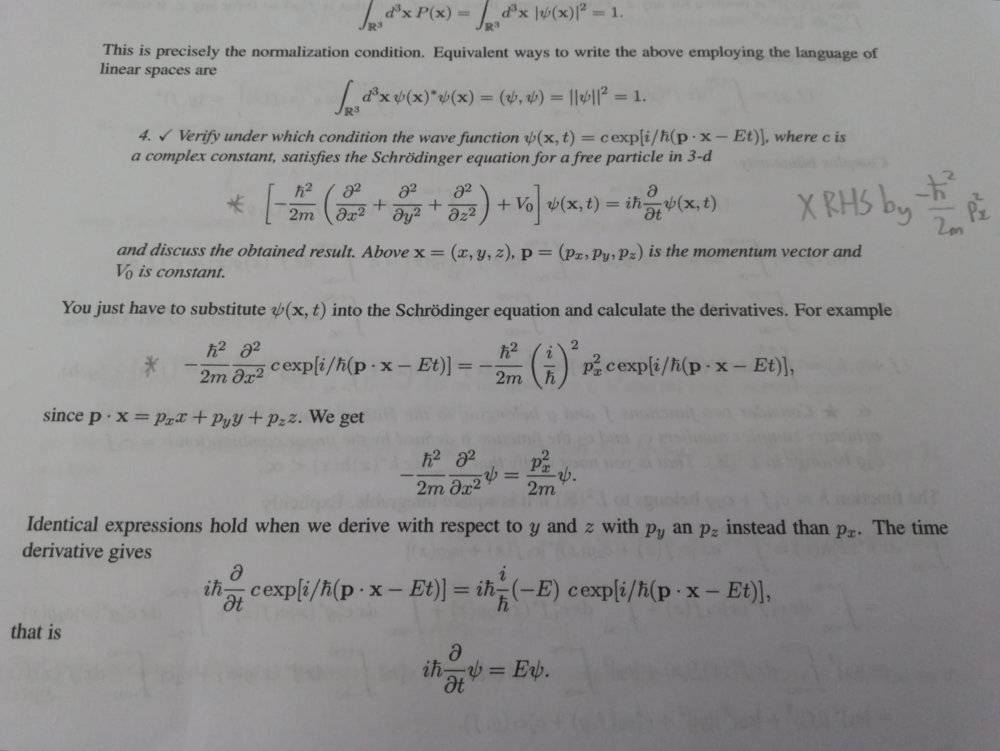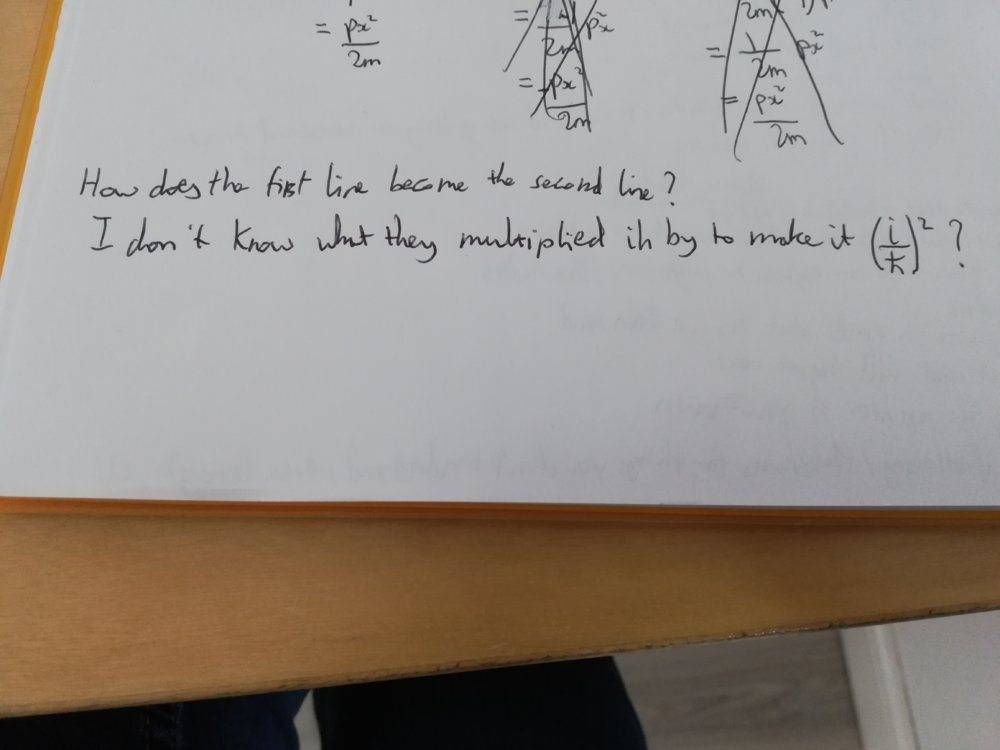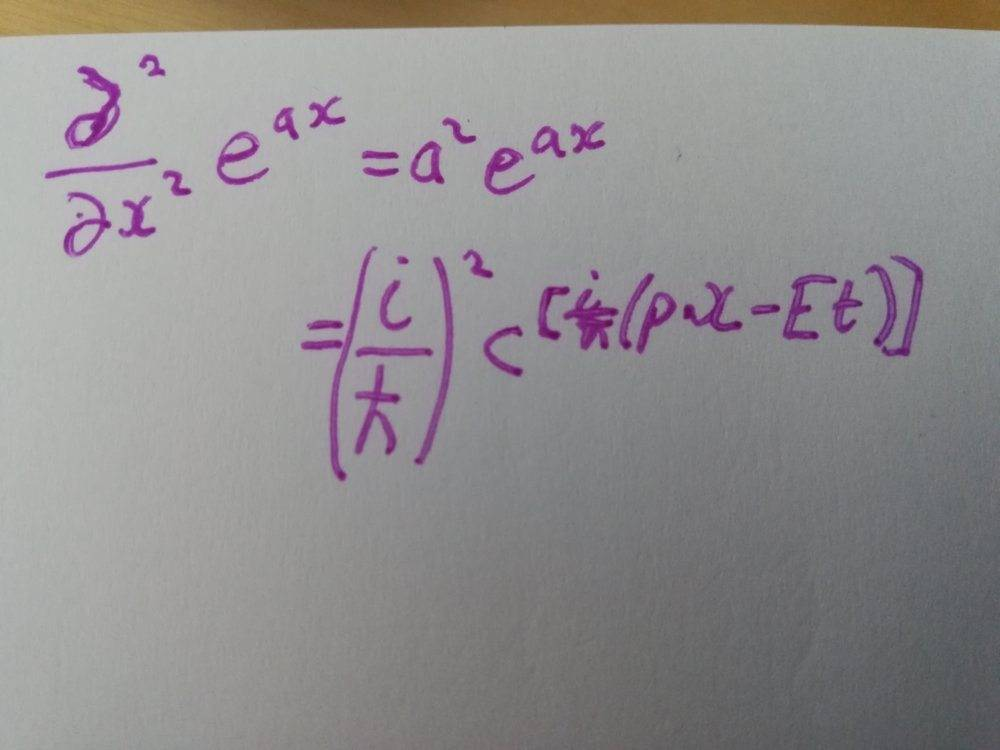# Schrodinger equation for a free particle in 3d space

I've got the solution to the question but I just need more detail. I can't work out the first step of the solution to the second step.That should read, I don't know what they multiplied ih-bar by to make it (i/h-bar)^2?

DrClaude
Mentor
I'm confused as to what you donate understand. Is it the appearance of ##(i/\hbar)^2## or it going away on the next line?

I'm confused as to what you donate understand. Is it the appearance of ##(i/\hbar)^2## or it going away on the next line?
Yeah, it's the appearance of ##(i/\hbar)^2##

DrClaude
Mentor
It comes from the derivative of the exponential.

$$\frac{\partial^2}{\partial x^2} e^{ax} = a^2 e^{ax}$$

It comes from the derivative of the exponential.

$$\frac{\partial^2}{\partial x^2} e^{ax} = a^2 e^{ax}$$
I only managed to get this. Can you show me the exact steps on how you ended up with the second line?And what are the values of a, e and x?

Nugatory
Mentor
And what are the values of a, e and x?
Did you intend to ask what ##e## is? It’s Euler’s number, and ##exp(a)## is another notation for ##e^a##. If you not already familiar with its properties you’re going to have to put some time into first-year differential and integral calculus before you can take on Schrodinger’s equation.

If that was just a slip of the typing fingers, go back to the ##\psi## suggested in the text, then rewrite the exponential of sums as a product of exponentials. Now you’ll be able to take the second derivative with respect to ##x## to get the ##(i/\hbar)^2## factor.

DrClaude
Mentor
To add to what @Nugatory said, ##a \in \mathbb{C}## is a constant and ##x## is the independent variable, as in the differentiation.